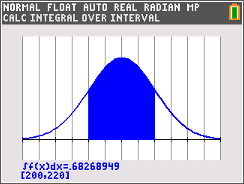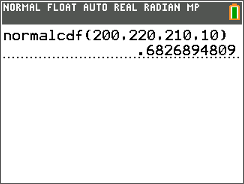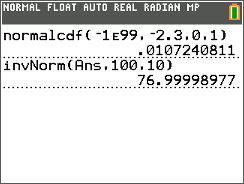### Mathematics lessons for IB® Diploma Programme

Applications and Interpretation | Probability and Statistics## Percentiles and Z-Score

### Activity Overview

Students calculate percentiles, z-scores and probabilities using normal distributions.

## Key Steps## Step 1

The activity begins with a review of the properties of normal curves along with a visual representation of the corresponding percentages. The worksheet then introduces z-scores and percentiles.## Step 2

Students are given a range of x-values and asked to find the probability (or percent) of data that fall within the interval. To find the answer, they will first use a graph and then follow up with numerical calculations.## Step 3

In the last two parts, students are to find the corresponding x-value given a percentile and then given a z-score and they are asked to find the corresponding percentile and x-value.